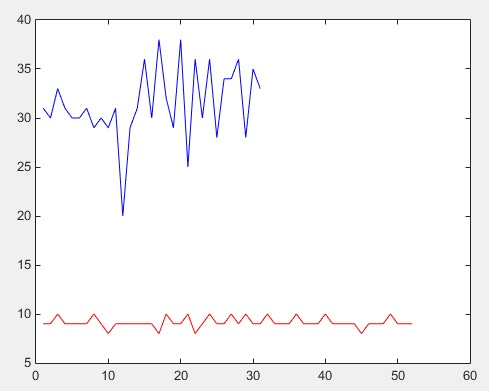1.蓝色部分表示一类数据，红色部分表示二类数据
2.数据都只有一个特征向量为y轴的值
3.x轴为数据在训练集中的下标

``````%训练样本

train_sample=[trainECG']; %21*25

%测试样本

test_sample=[testECG'];

%输出类别，建立HotCode

t1=[trainLabel'];

t2=[testLabel'];

train_result = ind2vec(t1);

test_result = ind2vec(t2);

net = newff(train_sample,train_result,4,{ 'tansig' 'purelin' } ,'traingdx');

net.trainParam.show=50; % 显示训练迭代过程

net.trainParam.epochs=15000; % 最大训练磁数

net.trainParam.goal=0.001; % 要求训练精度

net.trainParam.lr=0.02; % 学习率

net=init(net); %网络初始化

[net,tr]=train(net,train_sample,train_result); % 网络训练

result_sim=sim(net,test_sample); % 利用得到的神经网络仿真

result_sim_ind=vec2ind(result_sim);

correct=0;

for i=1:length(result_sim_ind)

if result_sim_ind(i)==t2(i);

correct=correct+1;

end

end

disp('正确率：');

correct / length(result_sim_ind)
``````